Algebra II : Graphing Radical Functions

Example Questions

Example Question #430 : Radicals

State the domain of the function: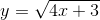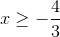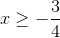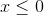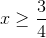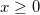Explanation:

Since the expression under the radical cannot be negative,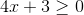.

Solve for x:This is the domain, or possiblevalues, for the function.

Example Question #431 : Radicals

Simplify the radical expression.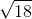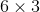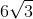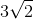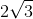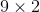Explanation:

To simplify the radical we need to break the number under the radical sign into its factors.

Since we are able to factor out 9 which is a perfect square, our radical becomes: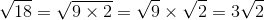Example Question #432 : Radicals

Simplify the radical expression.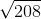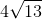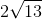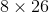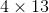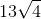Explanation:

To simplify the radical we need to factor the number under the radical sign.

Since we can factor out 16 which is a perfect square, the radical becomes: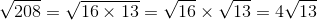Example Question #433 : Radicals

Simplify the radical expression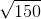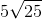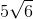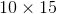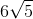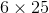Explanation:

To simplify the radical we need to factor the number under the radical sign.

Since we can factor out a 25 which is a perfect square, the radical becomes: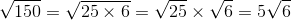Example Question #434 : Radicals

Simplify the radical expression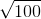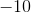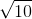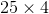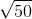Explanation:

To simplify the radical we need to first factor the number under the radical sign.

Since we can factor out a 25 and 4 which are perfect squares, our radical becomes: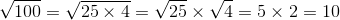Example Question #435 : Radicals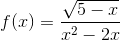Which of the following choices correctly describes the domain of the graph of the function?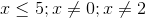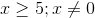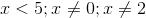All real numbers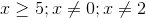Explanation:

Because the term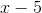inside the radical in the numerator has to be greater than or equal to zero, there is a restriction on our domain; to describe this restriction we solve the inequality for: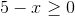Addto both sides, and the resulting inequality is: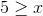or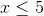Next, we consider the denominator, a quadratic; we know that we cannot divide by zero, so we must find x-values that make the denominator equal zero, and exclude them from our domain: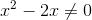We factor anout of bothand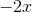: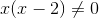Finding the zeroes of our expression leaves us with: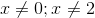Therefore, there are 3 restrictions on the domain of the function:All Algebra II Resources